Make nice scatter plots easily.

## Usage

nice_scatter(
data,
predictor,
response,
xtitle = predictor,
ytitle = response,
has.points = TRUE,
has.jitter = FALSE,
alpha = 0.7,
has.line = TRUE,
method = "lm",
has.confband = FALSE,
has.fullrange = FALSE,
has.linetype = FALSE,
has.shape = FALSE,
xmin,
xmax,
xby = 1,
ymin,
ymax,
yby = 1,
has.legend = FALSE,
legend.title = "",
group = NULL,
colours = "#619CFF",
groups.order = "none",
groups.labels = NULL,
groups.alpha = NULL,
has.r = FALSE,
r.x = Inf,
r.y = -Inf,
has.p = FALSE,
p.x = Inf,
p.y = -Inf
)

## Arguments

data

The data frame.

predictor

The independent variable to be plotted.

response

The dependent variable to be plotted.

xtitle

An optional y-axis label, if desired.

ytitle

An optional x-axis label, if desired.

has.points

Whether to plot the individual observations or not.

has.jitter

Alternative to has.points. "Jitters" the observations to avoid overlap (overplotting). Use one or the other, not both.

alpha

The desired level of transparency.

has.line

Whether to plot the regression line(s).

method

Which method to use for the regression line, either "lm" (default) or "loess".

has.confband

Logical. Whether to display the confidence band around the slope.

has.fullrange

Logical. Whether to extend the slope beyond the range of observations.

has.linetype

Logical. Whether to change line types as a function of group.

has.shape

Logical. Whether to change shape of observations as a function of group.

xmin

The minimum score on the x-axis scale.

xmax

The maximum score on the x-axis scale.

xby

How much to increase on each "tick" on the x-axis scale.

ymin

The minimum score on the y-axis scale.

ymax

The maximum score on the y-axis scale.

yby

How much to increase on each "tick" on the y-axis scale.

has.legend

Logical. Whether to display the legend or not.

legend.title

The desired legend title.

group

The group by which to plot the variable

colours

Desired colours for the plot, if desired.

groups.order

Specifies the desired display order of the groups on the legend. Either provide the levels directly, or a string: "increasing" or "decreasing", to order based on the average value of the variable on the y axis, or "string.length", to order from the shortest to the longest string (useful when working with long string names). "Defaults to "none".

groups.labels

Changes groups names (labels). Note: This applies after changing order of level.

groups.alpha

The manually specified transparency desired for the groups slopes. Use only when plotting groups separately.

has.r

Whether to display the correlation coefficient, the r-value.

r.x

The x-axis coordinates for the r-value.

r.y

The y-axis coordinates for the r-value.

has.p

Whether to display the p-value.

p.x

The x-axis coordinates for the p-value.

p.y

The y-axis coordinates for the p-value.

## Value

A scatter plot of class ggplot.

Visualize group differences via violin plots: nice_violin. Tutorial: https://rempsyc.remi-theriault.com/articles/scatter

## Examples

# Make the basic plot
nice_scatter(
data = mtcars,
predictor = "wt",
response = "mpg"
)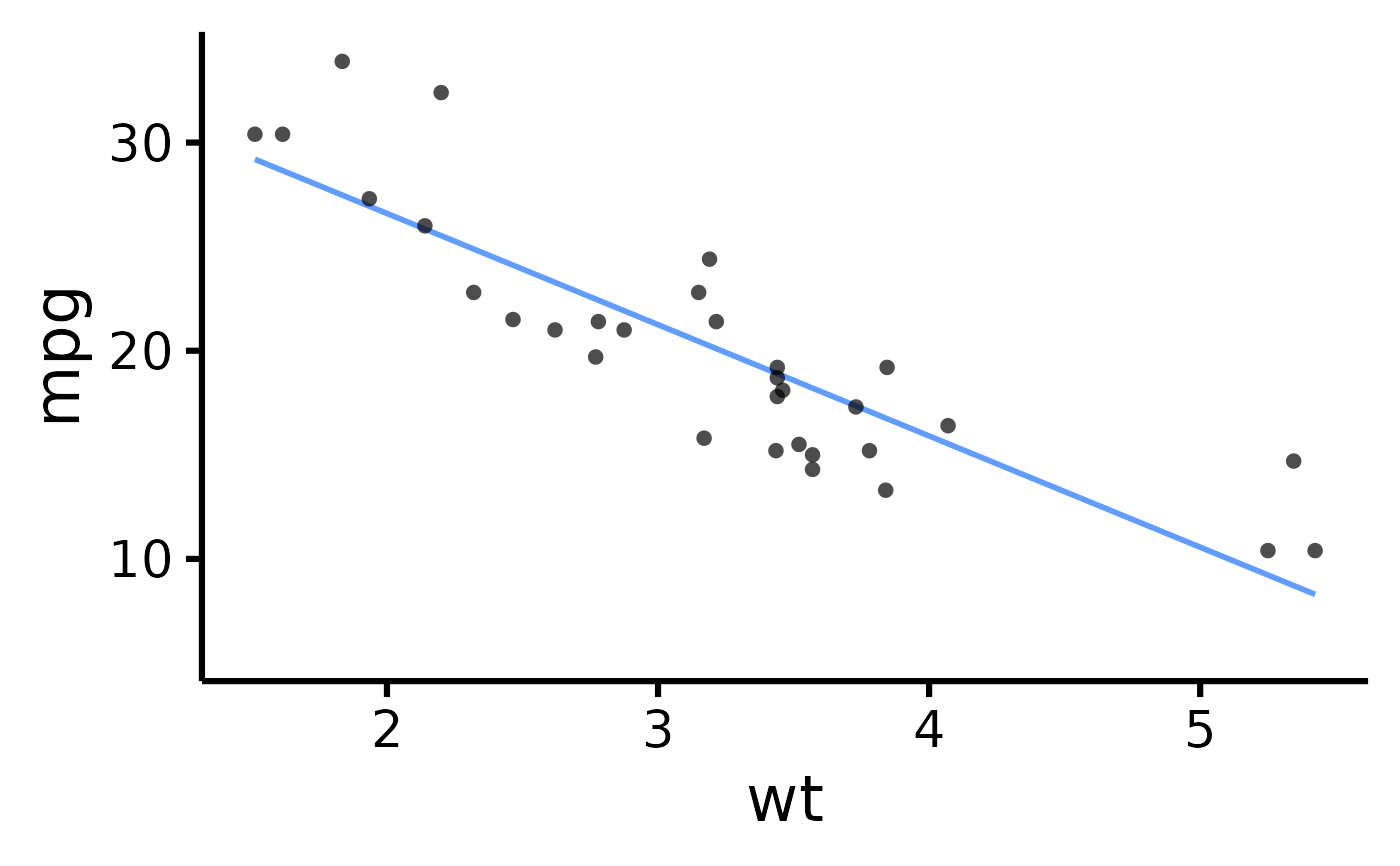# \donttest{
.old_wd <- setwd(tempdir())
# Save a high-resolution image file to specified directory
ggplot2::ggsave("nicescatterplothere.pdf", width = 7,
height = 7, unit = "in", dpi = 300
) # change for your own desired path
setwd(.old_wd)
# Change x- and y- axis labels
nice_scatter(
data = mtcars,
predictor = "wt",
response = "mpg",
ytitle = "Miles/(US) gallon",
xtitle = "Weight (1000 lbs)"
)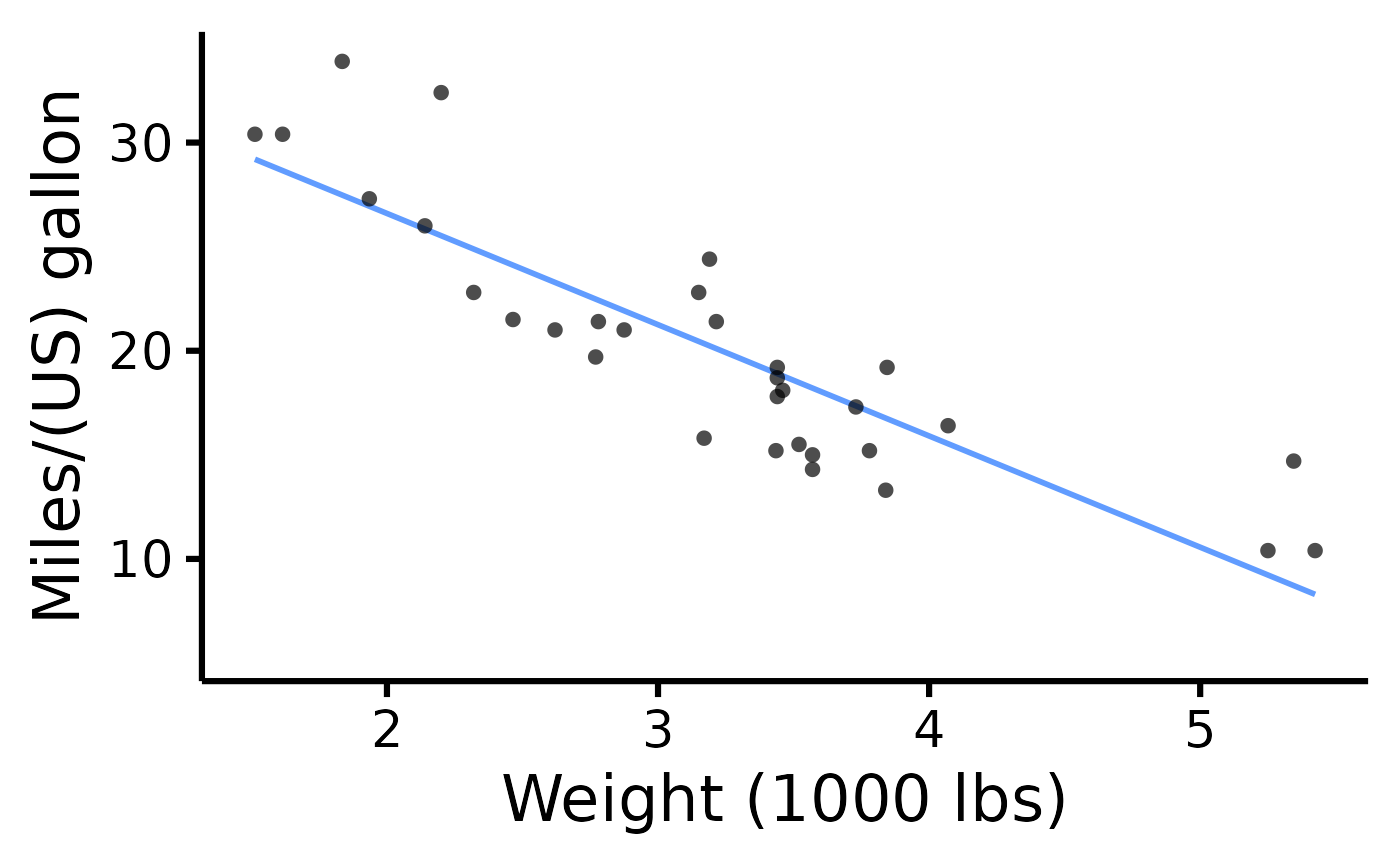# Have points "jittered", loess method
nice_scatter(
data = mtcars,
predictor = "wt",
response = "mpg",
has.jitter = TRUE,
method = "loess"
)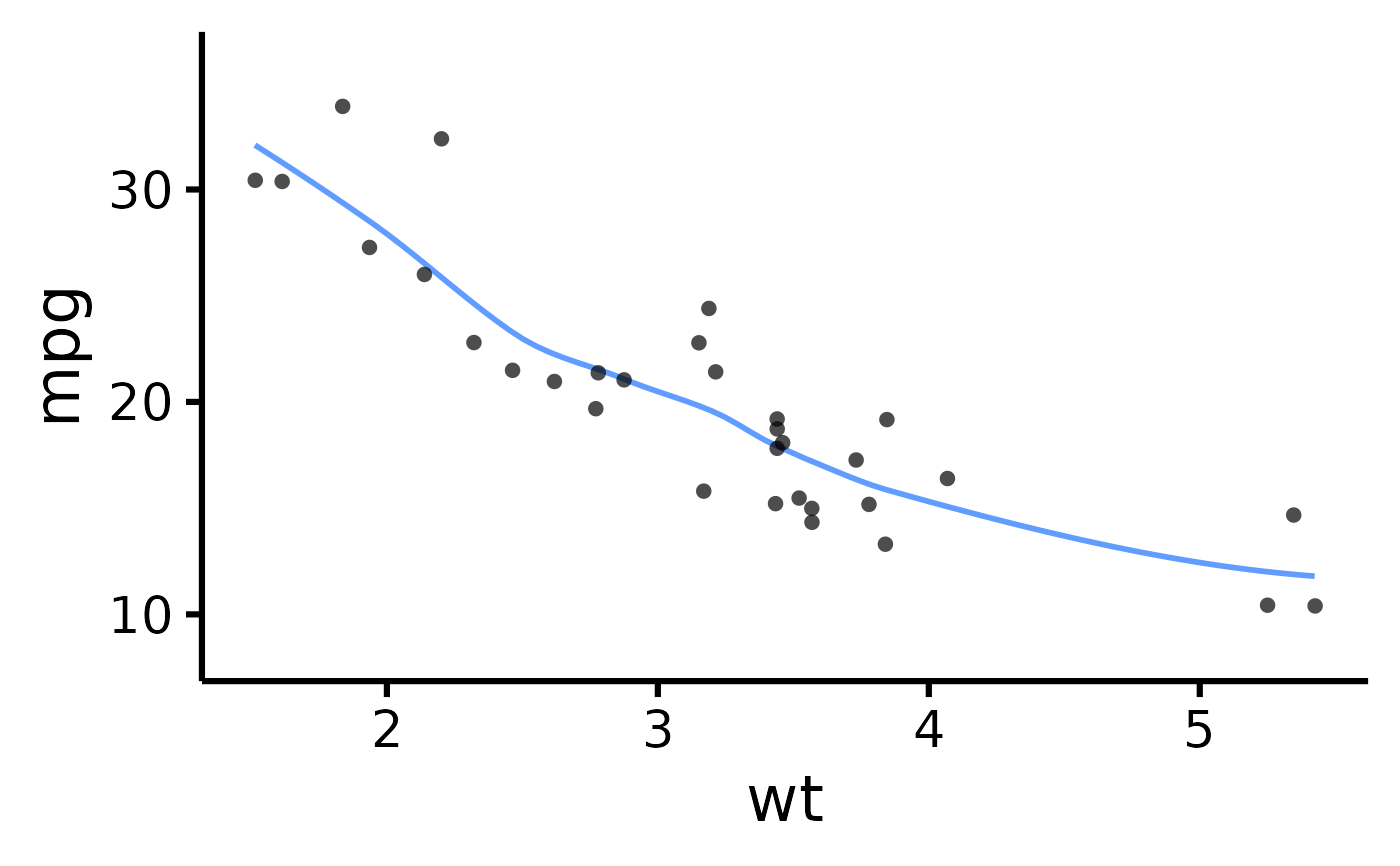# Change the transparency of the points
nice_scatter(
data = mtcars,
predictor = "wt",
response = "mpg",
alpha = 1
)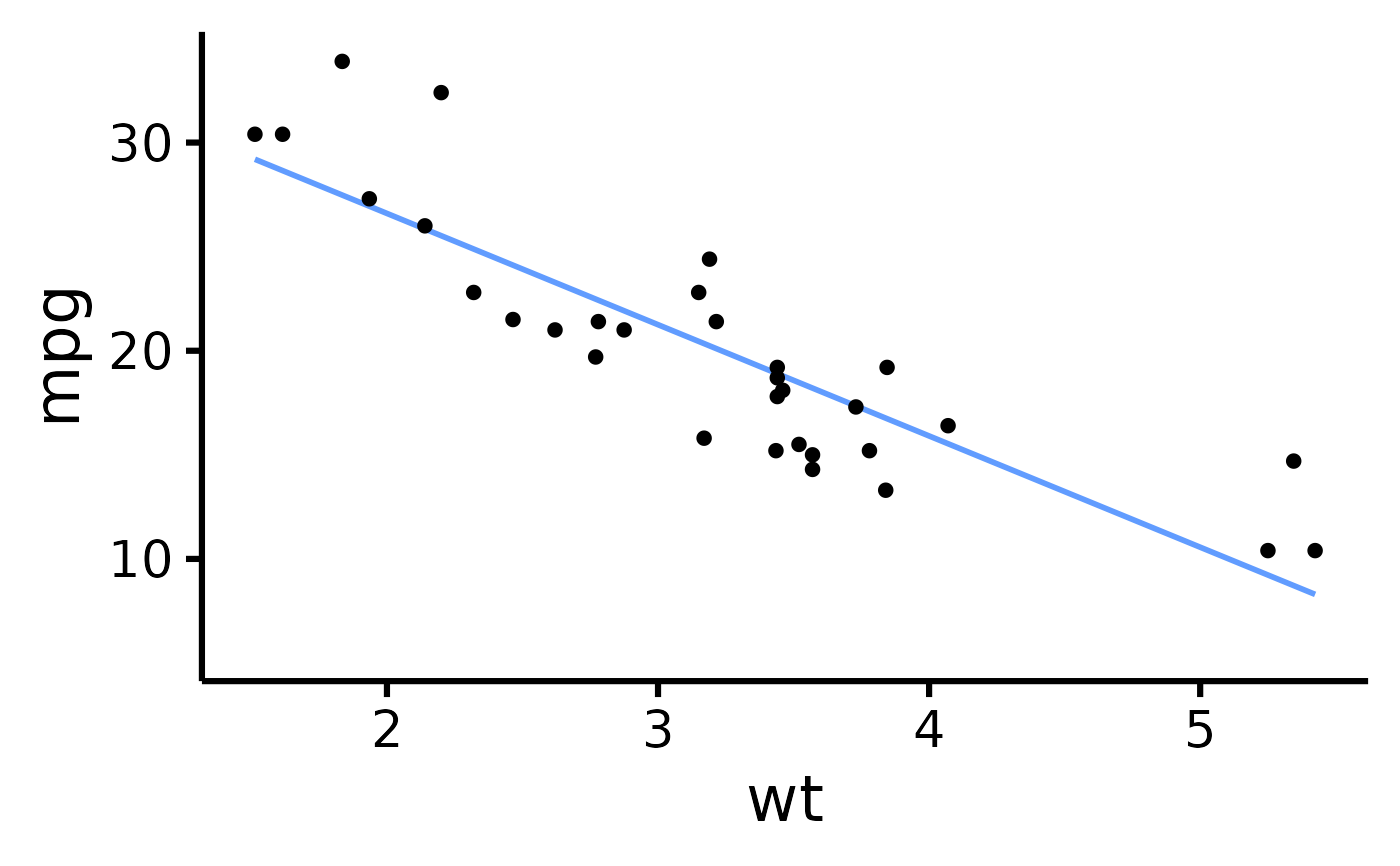# Remove points
nice_scatter(
data = mtcars,
predictor = "wt",
response = "mpg",
has.points = FALSE,
has.jitter = FALSE
)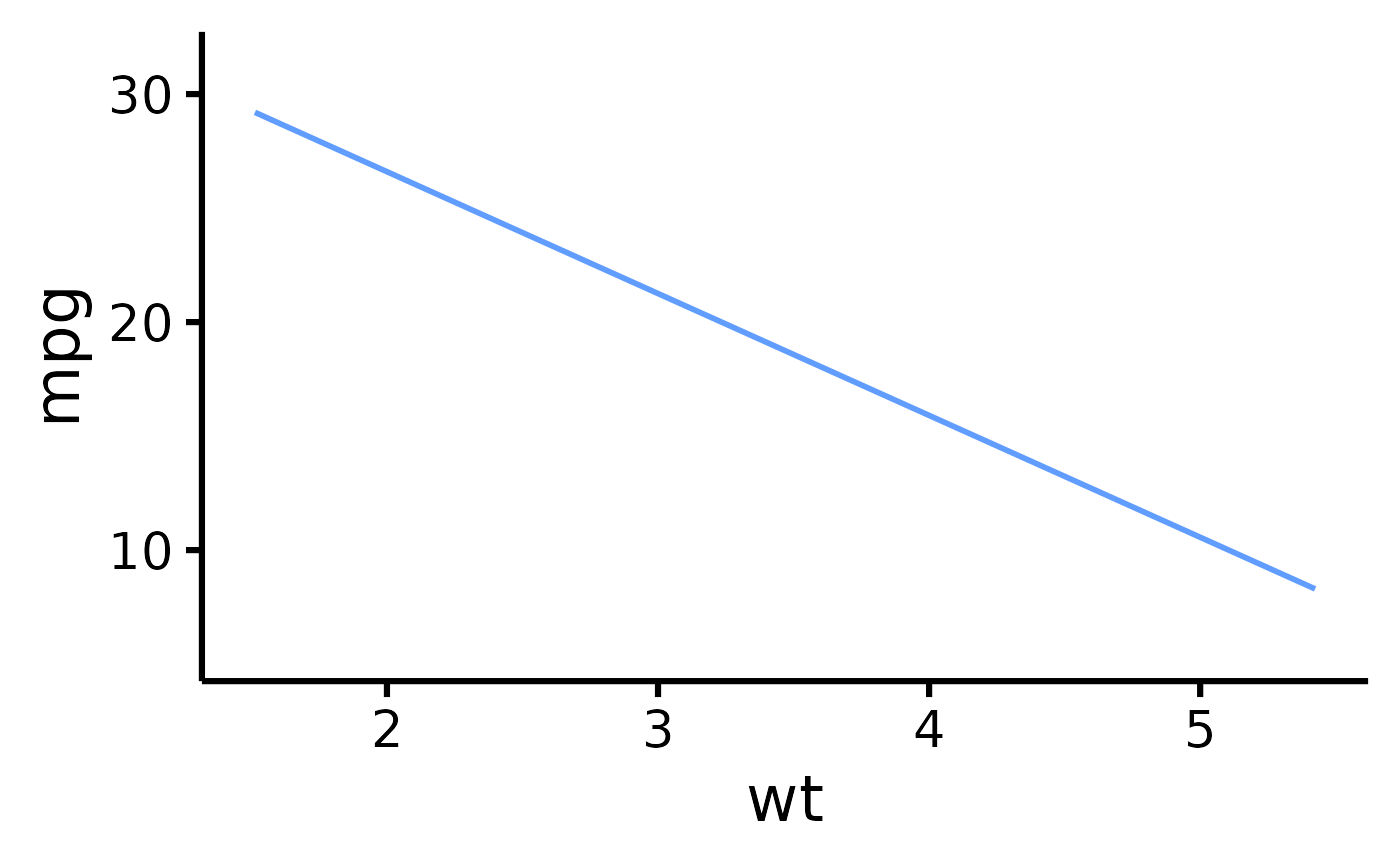nice_scatter(
data = mtcars,
predictor = "wt",
response = "mpg",
has.confband = TRUE
)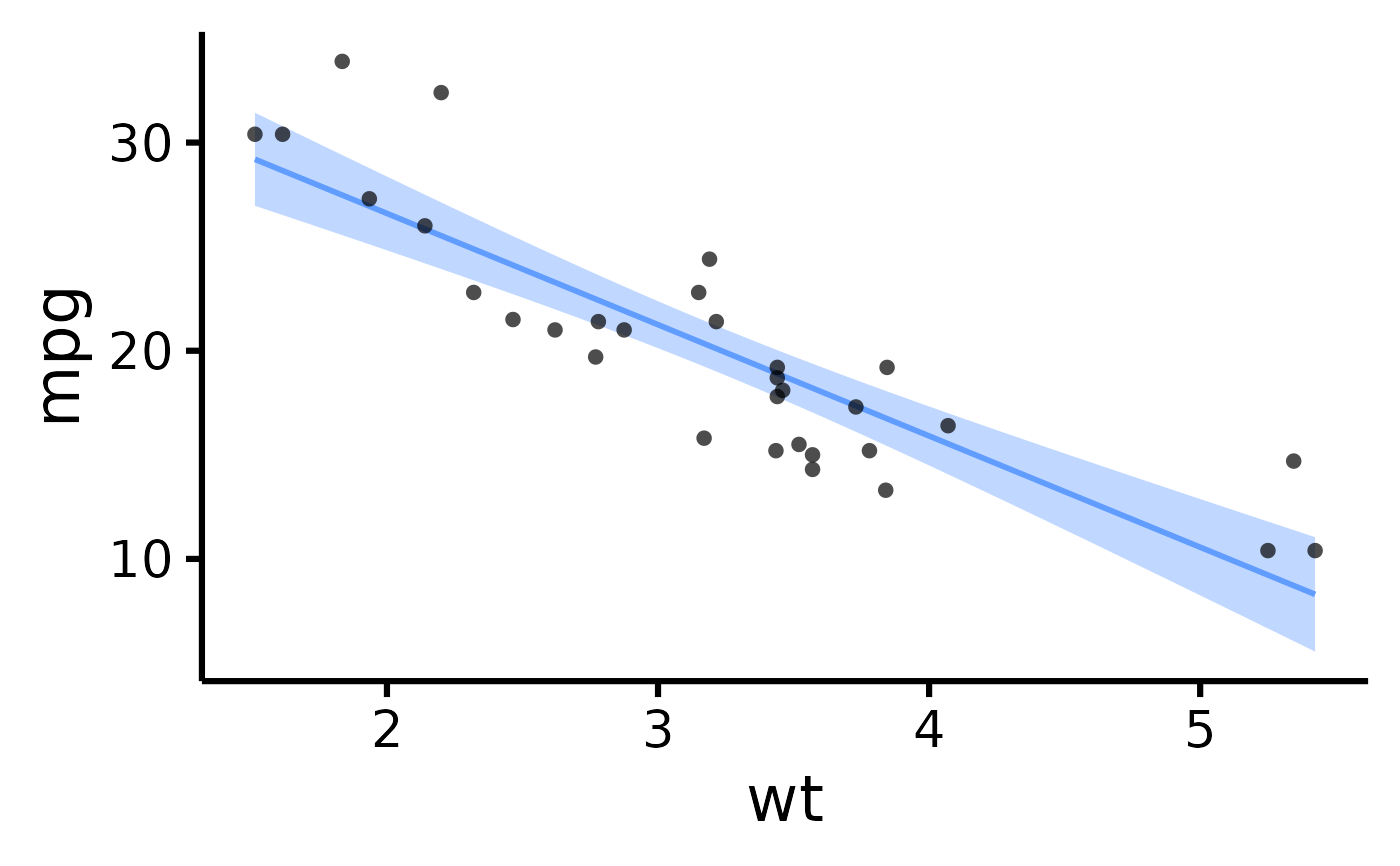# Set x- and y- scales manually
nice_scatter(
data = mtcars,
predictor = "wt",
response = "mpg",
xmin = 1,
xmax = 6,
xby = 1,
ymin = 10,
ymax = 35,
yby = 5
)
#> Warning: Removed 7 rows containing missing values (geom_line()).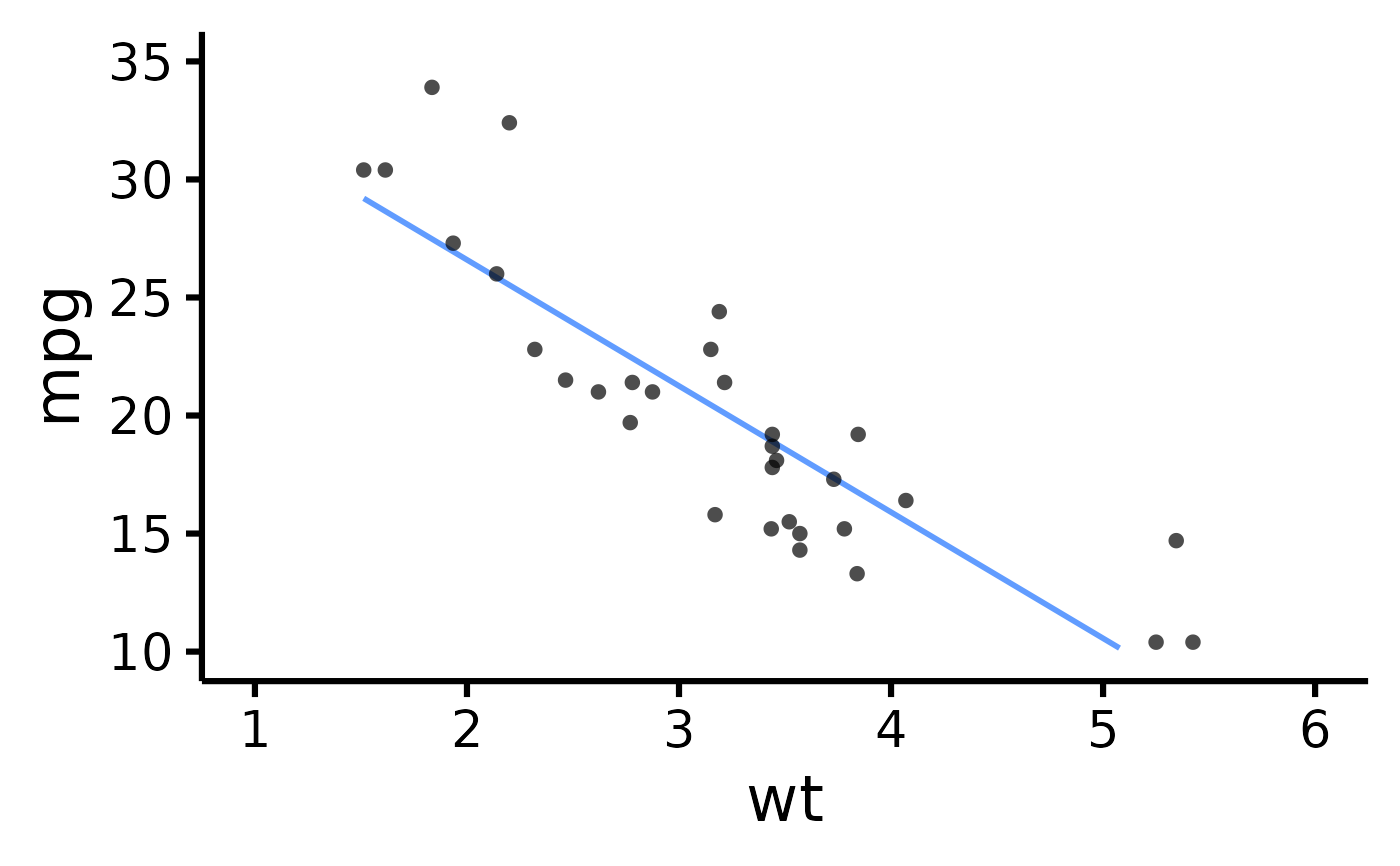# Change plot colour
nice_scatter(
data = mtcars,
predictor = "wt",
response = "mpg",
colours = "blueviolet"
)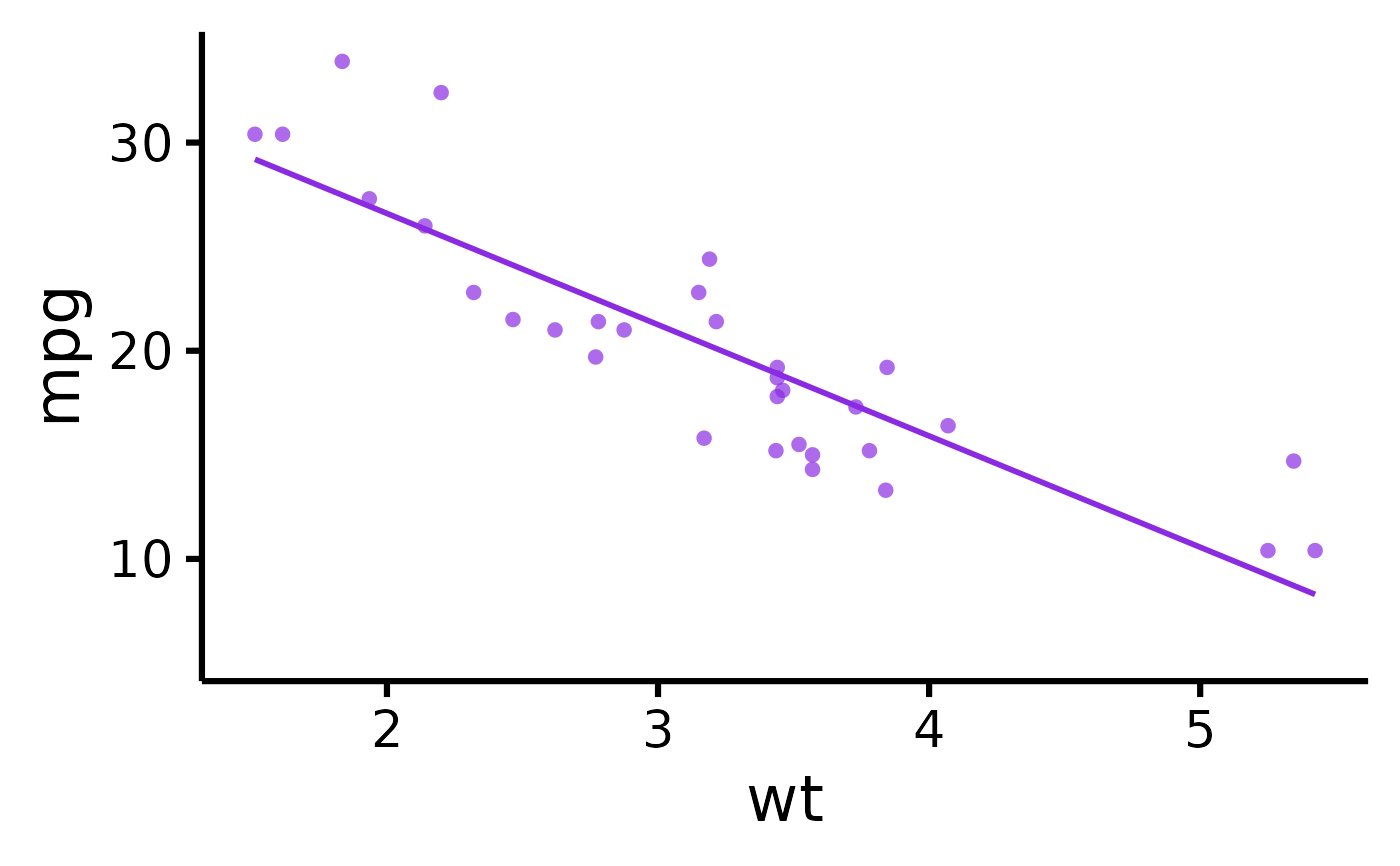# Add correlation coefficient to plot and p-value
nice_scatter(
data = mtcars,
predictor = "wt",
response = "mpg",
has.r = TRUE,
has.p = TRUE
)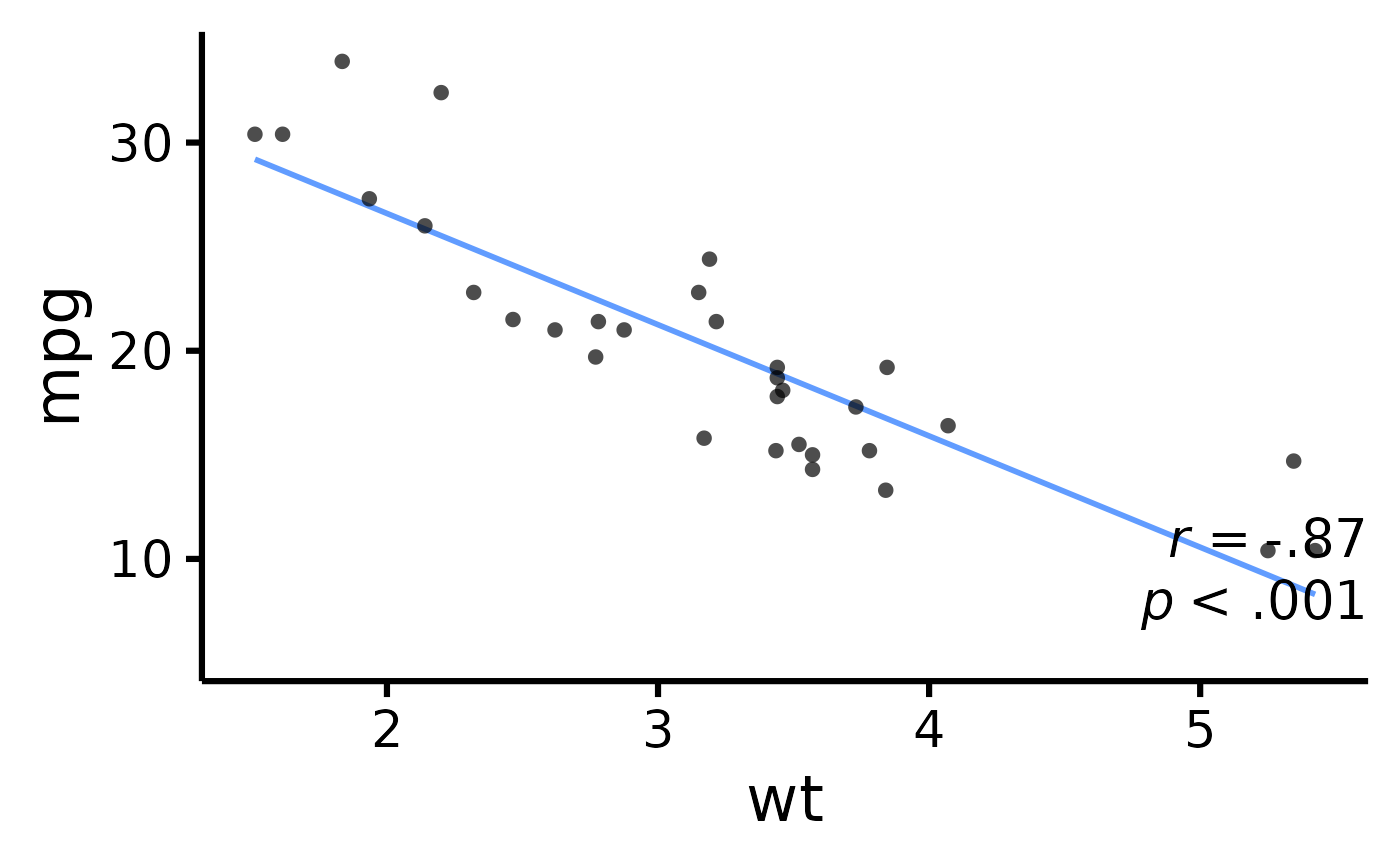# Change location of correlation coefficient or p-value
nice_scatter(
data = mtcars,
predictor = "wt",
response = "mpg",
has.r = TRUE,
r.x = 4,
r.y = 25,
has.p = TRUE,
p.x = 5,
p.y = 20
)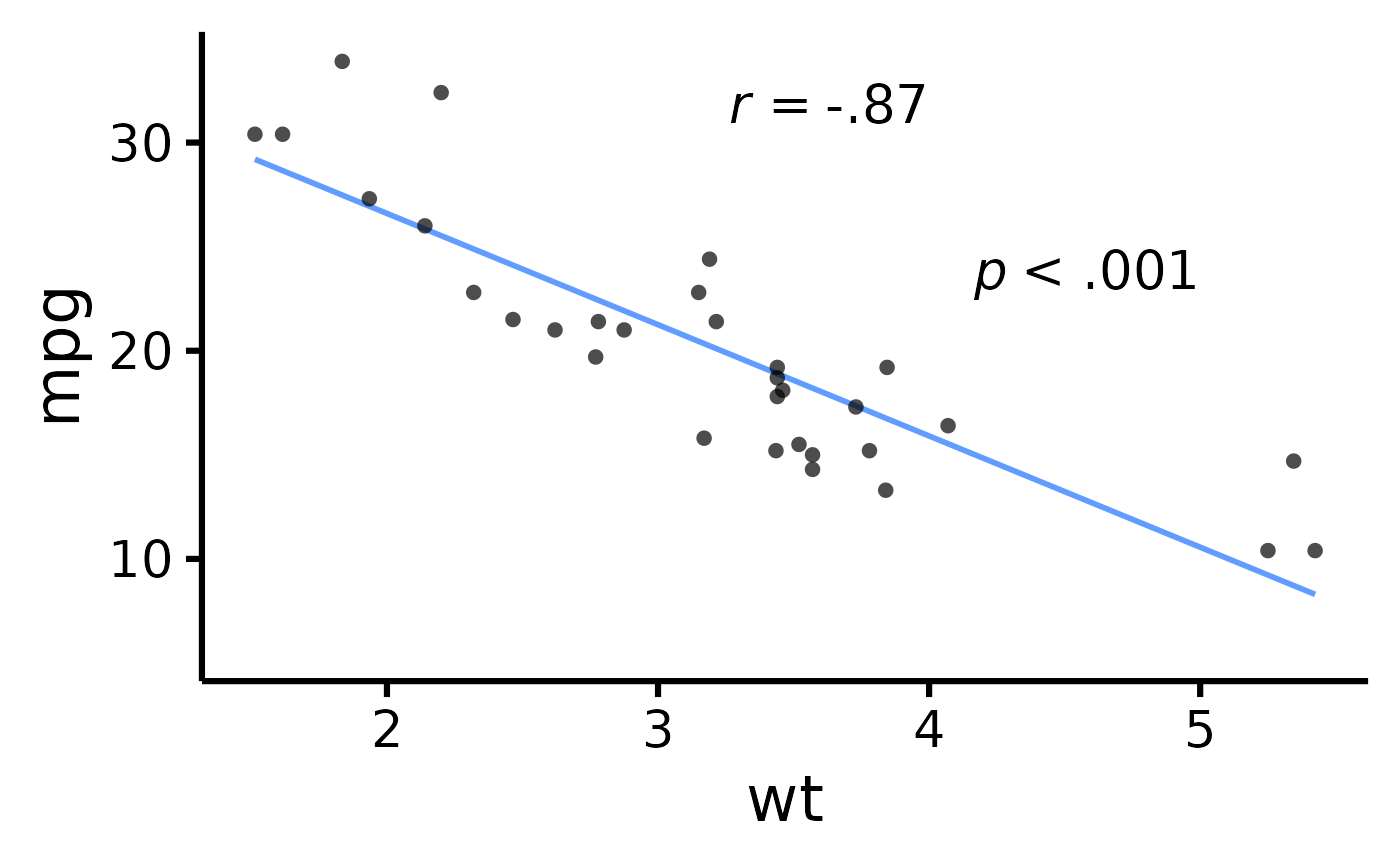# Plot by group
nice_scatter(
data = mtcars,
predictor = "wt",
response = "mpg",
group = "cyl"
)# Use full range on the slope/confidence band
nice_scatter(
data = mtcars,
predictor = "wt",
response = "mpg",
group = "cyl",
has.fullrange = TRUE
)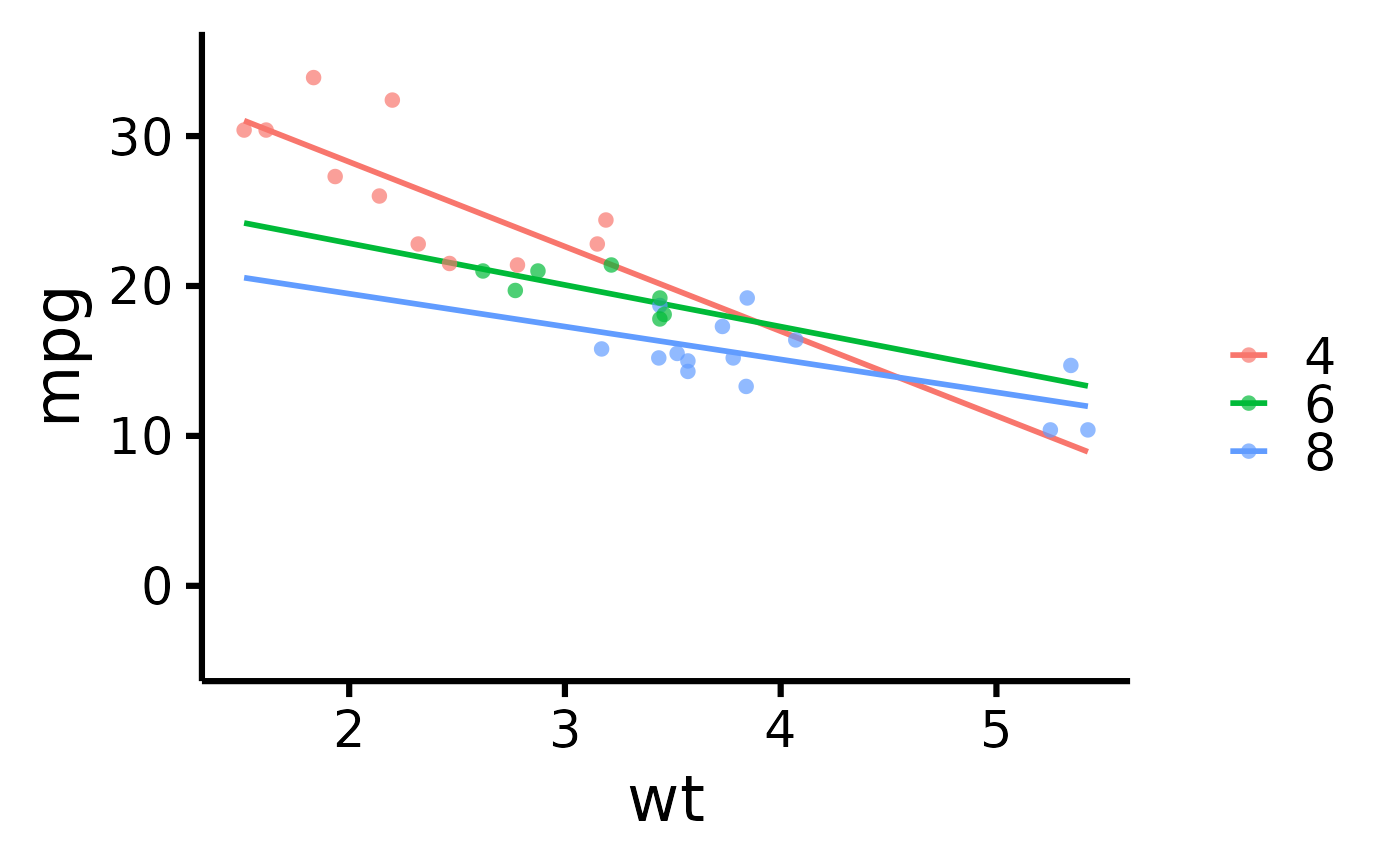# Remove lines
nice_scatter(
data = mtcars,
predictor = "wt",
response = "mpg",
group = "cyl",
has.line = FALSE
)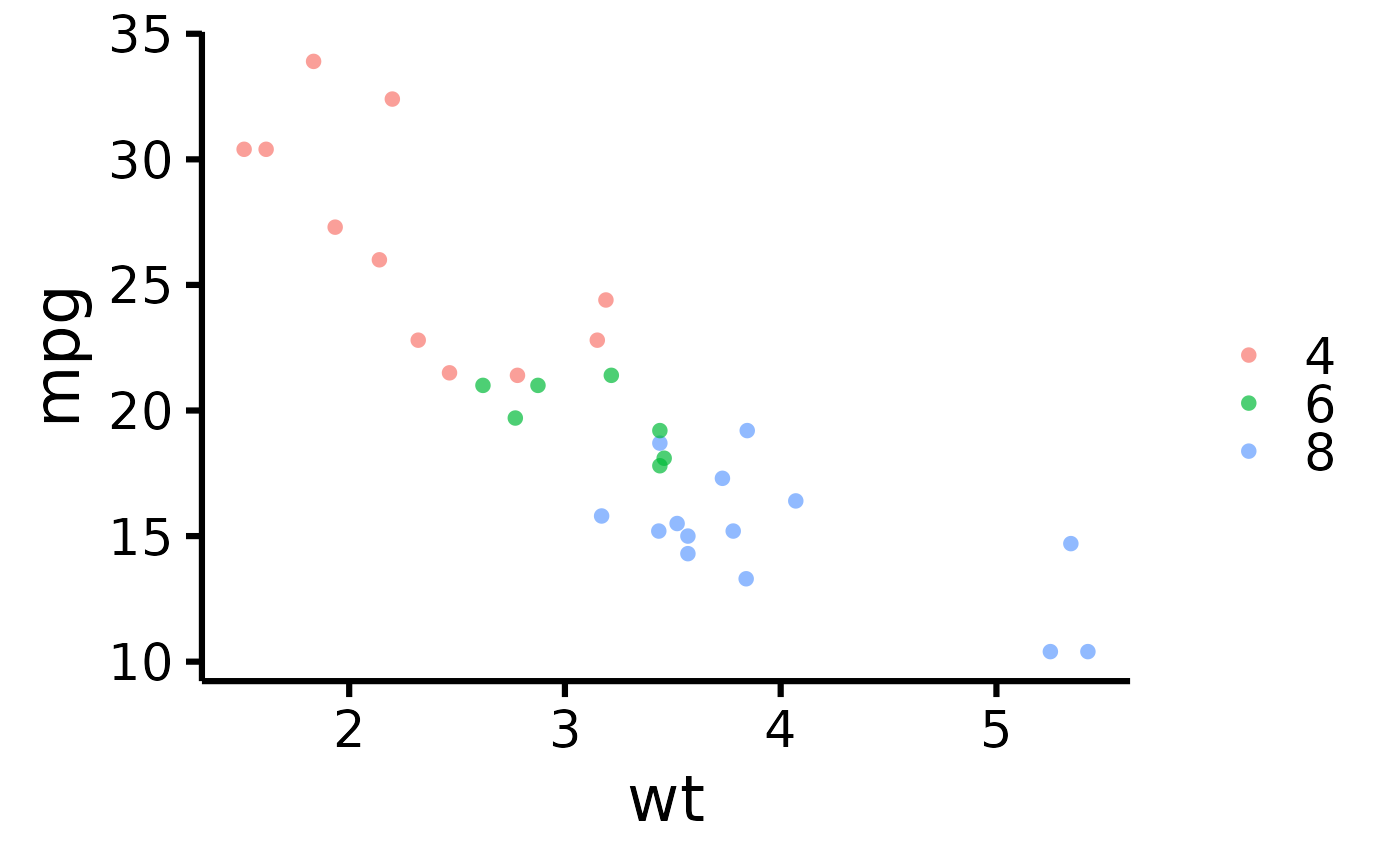# Change order of labels on the legend
nice_scatter(
data = mtcars,
predictor = "wt",
response = "mpg",
group = "cyl",
groups.order = c(8, 4, 6)
)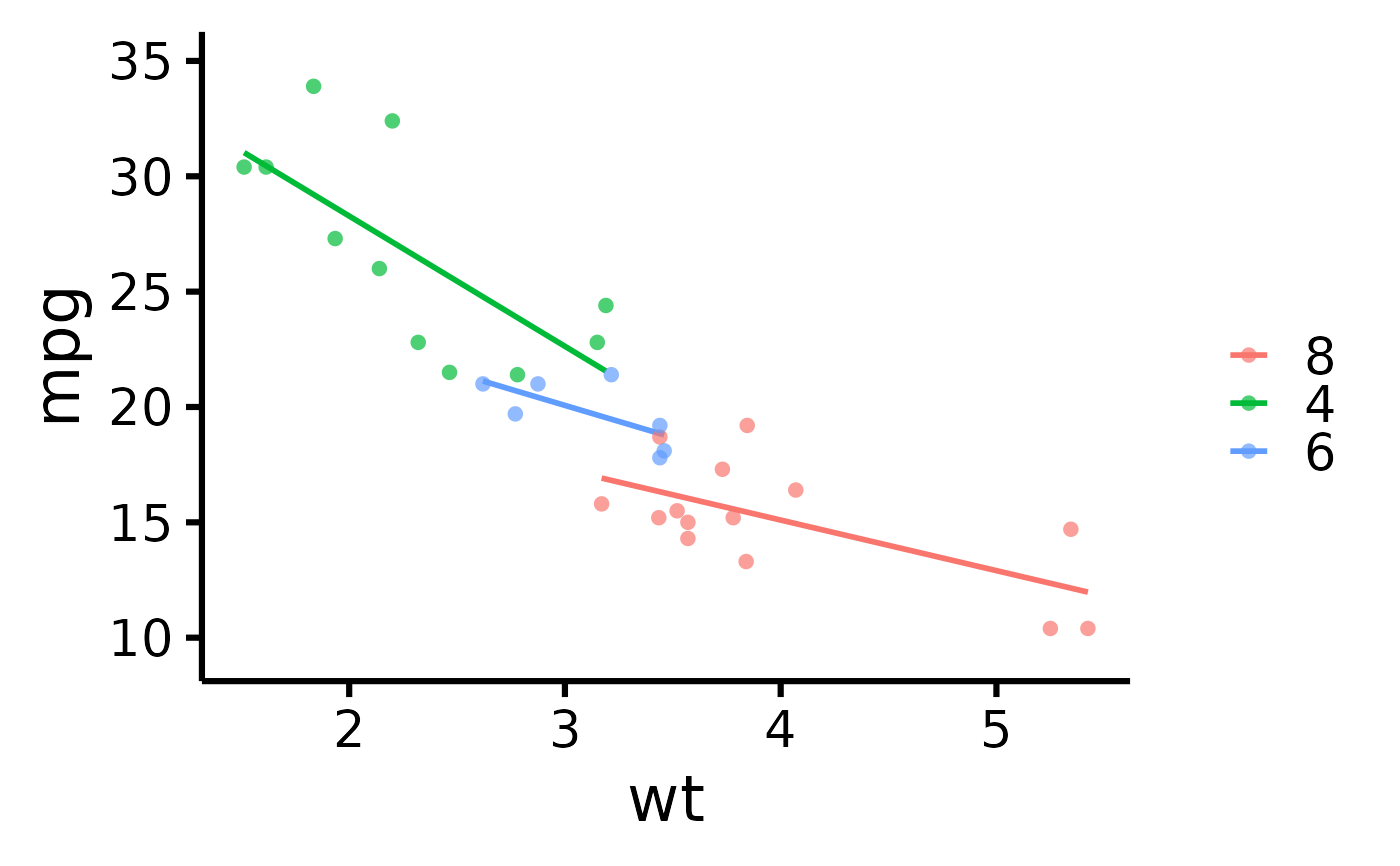# Change legend labels
nice_scatter(
data = mtcars,
predictor = "wt",
response = "mpg",
group = "cyl",
groups.labels = c("Weak", "Average", "Powerful")
)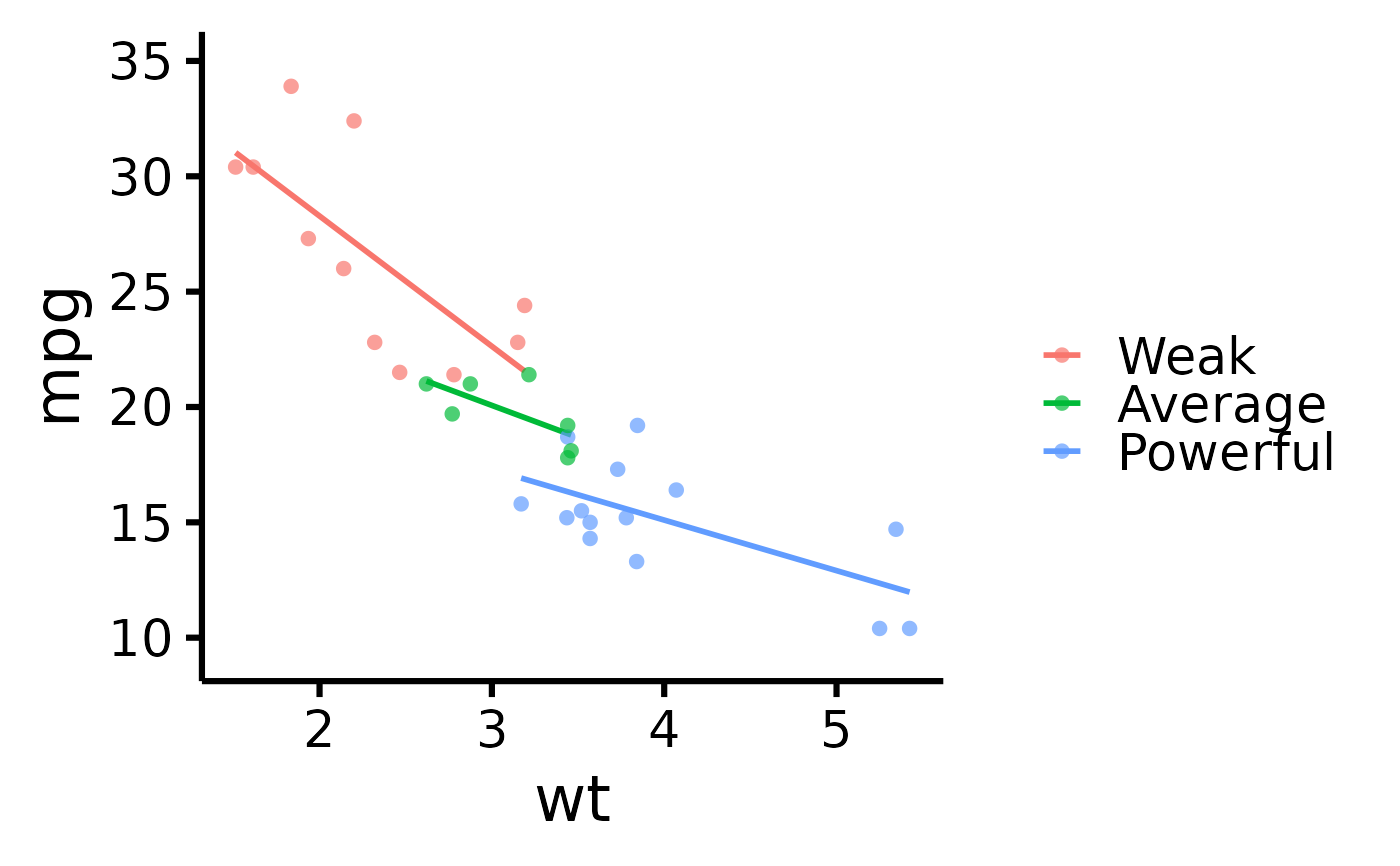# Warning: This applies after changing order of level

# Add a title to legend
nice_scatter(
data = mtcars,
predictor = "wt",
response = "mpg",
group = "cyl",
legend.title = "cylinders"
)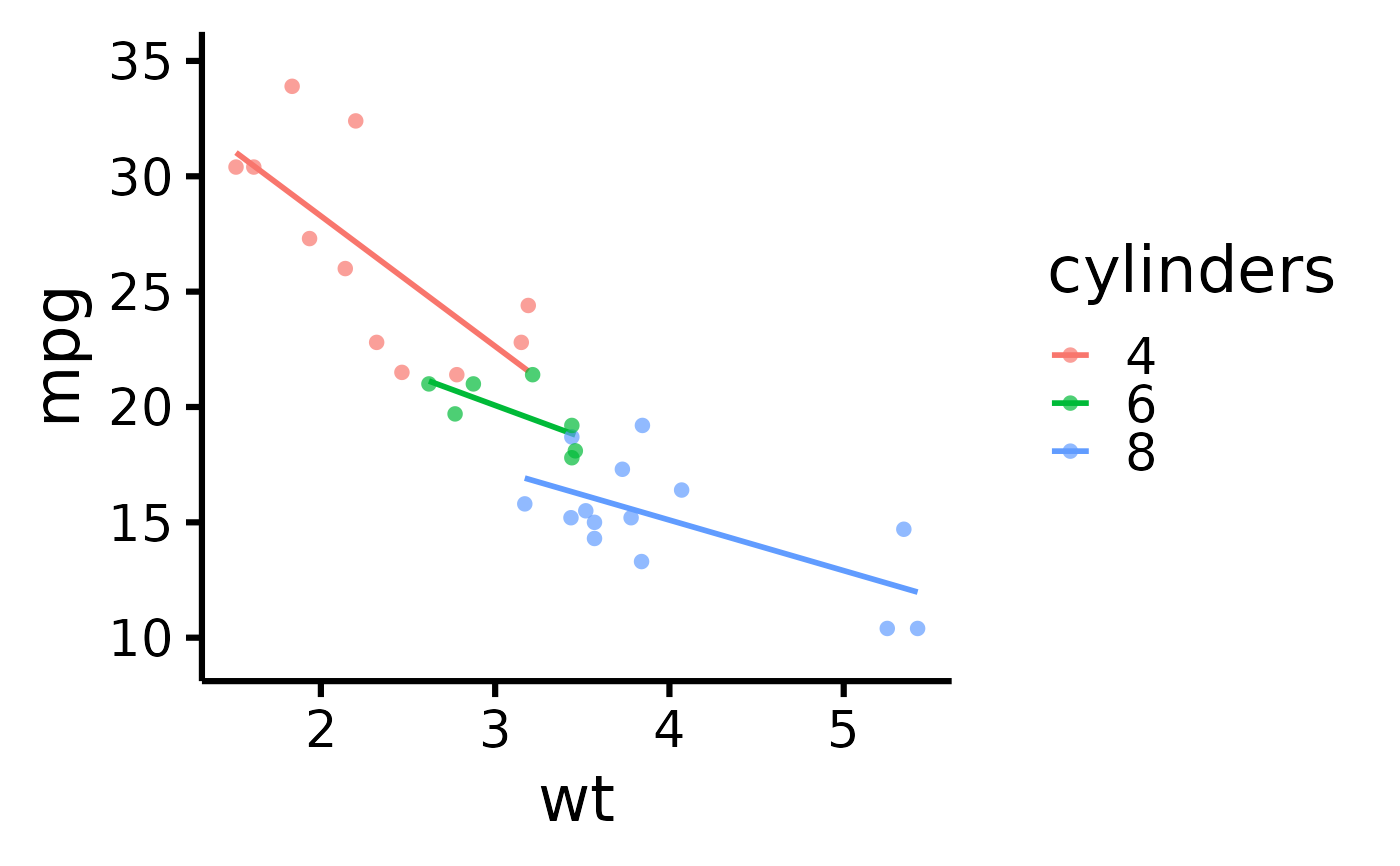# Plot by group + manually specify colours
nice_scatter(
data = mtcars,
predictor = "wt",
response = "mpg",
group = "cyl",
colours = c("burlywood", "darkgoldenrod", "chocolate")
)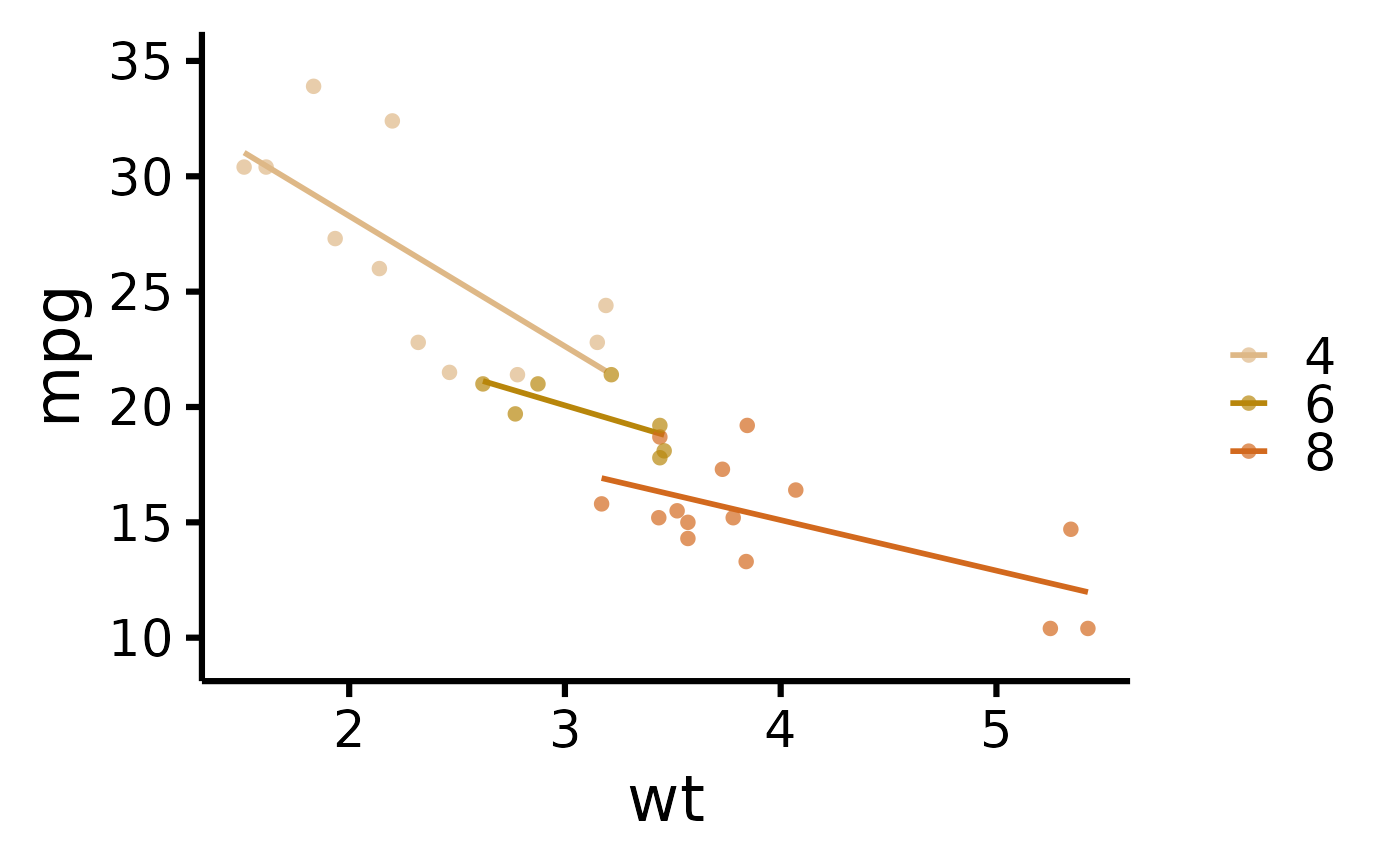# Plot by group + use different line types for each group
nice_scatter(
data = mtcars,
predictor = "wt",
response = "mpg",
group = "cyl",
has.linetype = TRUE
)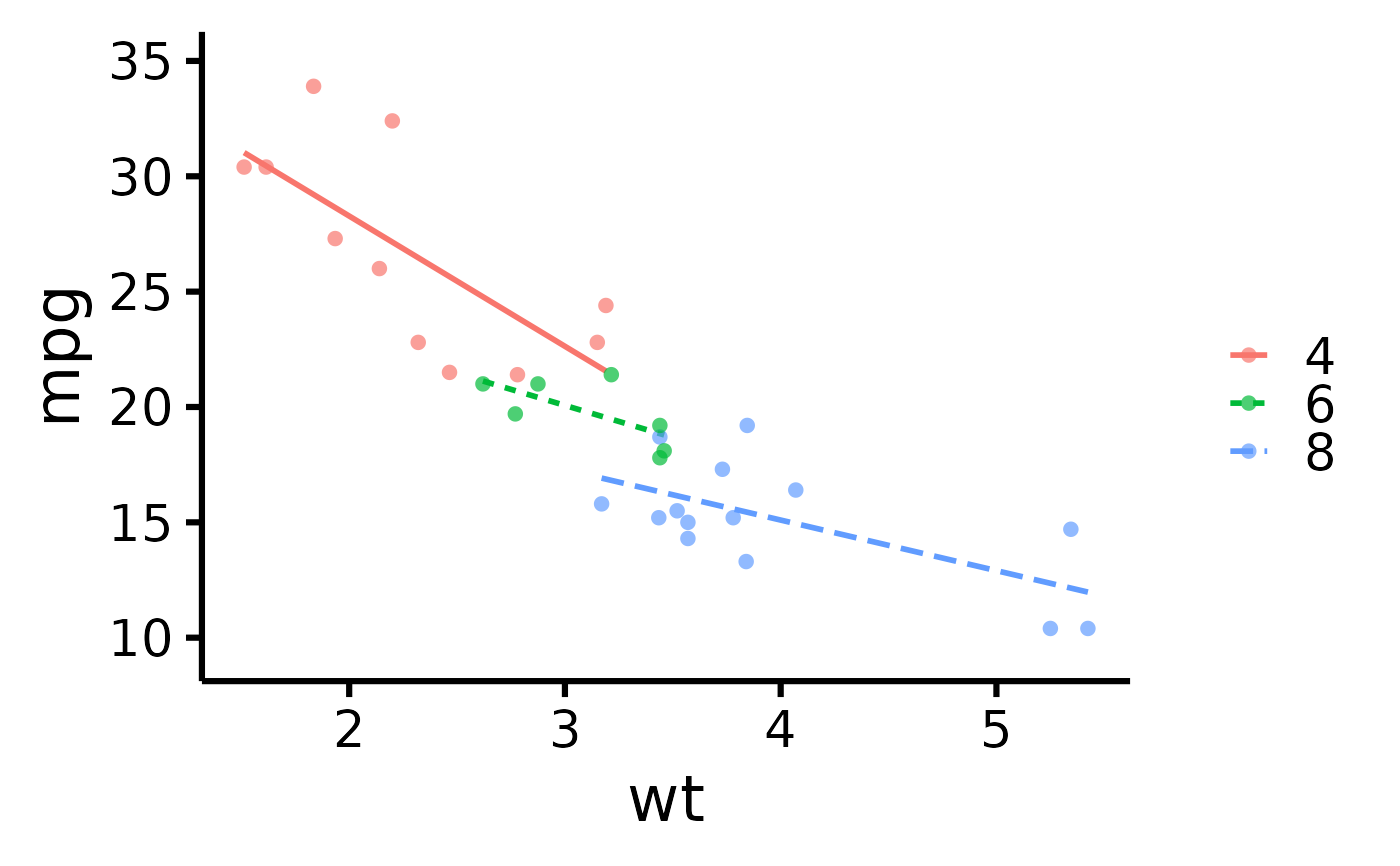# Plot by group + use different point shapes for each group
nice_scatter(
data = mtcars,
predictor = "wt",
response = "mpg",
group = "cyl",
has.shape = TRUE
)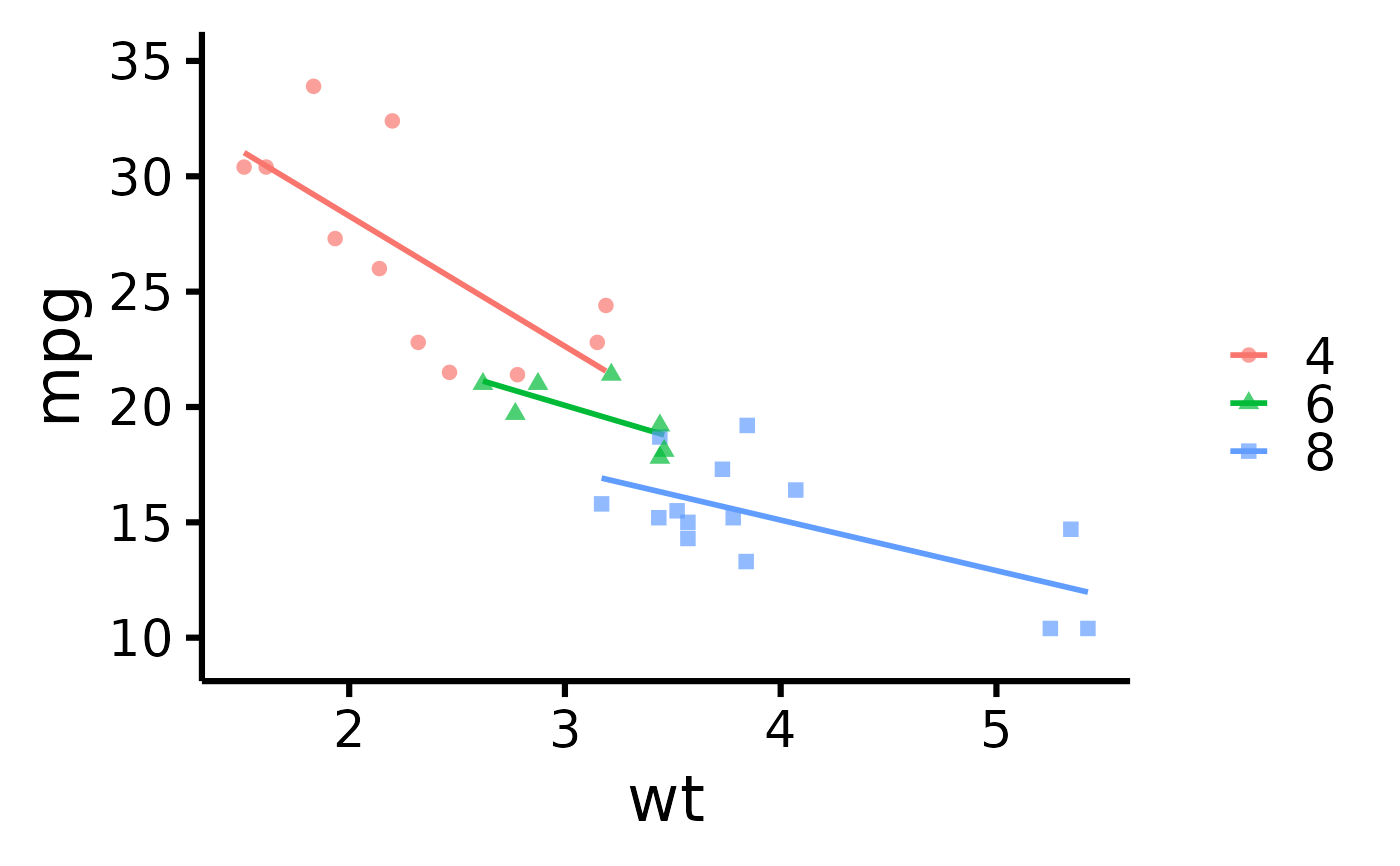# }